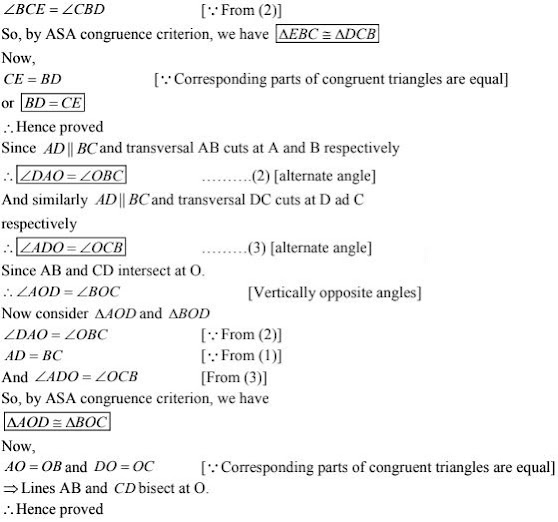# Chapter 10 Congruent Triangles RD Sharma Solutions Exercise 10.2 Class 9 Maths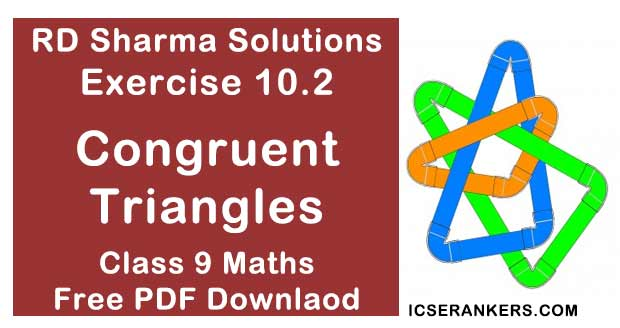Chapter Name RD Sharma Chapter 10 Congruent Triangles Exercise 10.2 Book Name RD Sharma Mathematics for Class 10 Other Exercises Exercise 10.1Exercise 10.3Exercise 10.4Exercise 10.5Exercise 10.6 Related Study NCERT Solutions for Class 10 Maths

### Exercise 10.2 Solutions

1. In Fig. 10.40, it is given that RT = TS, ∠1 = 2∠2 and ∠4 = 2∠3. Prove that ΔRBT ≅ ΔSAT.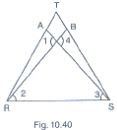Solution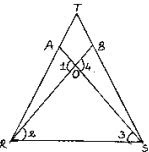In the figure given that
RT = TS  ...(1)
∠1 = 2∠2  ...(2)
and ∠4 = 2∠3 ...(3)
and given to prove ΔRBT ≅ ΔSAT
Let the point of intersection of RB and SA be denoted by O
Since RB and SA intersect at O.
∴ ∠AOR = ∠BOS  [Vertically opposite angles]
⇒ ∠1 = ∠4
⇒ 2∠2 = 2∠3  [From (2) and (3)]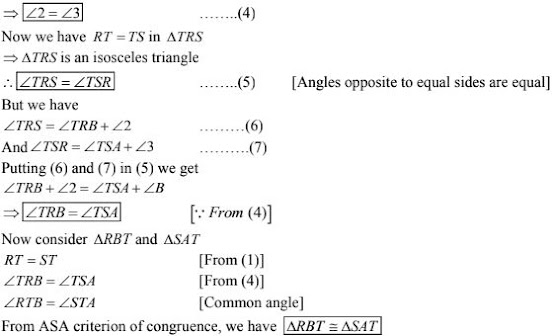2. Two lines AB and CD intersect at O such that BC is equal and parallel to AD. Prove that the lines AB and CD bisect at O.
Solution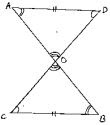Given that lines AB and CD intersect at O
We have to prove that AB and CD bisect at O.
To prove this first we have to prove that ΔAOD ≅ ΔBOC

3. BD and CE are bisectors of ∠B and ∠C of an isosceles ΔABC with AB = AC. Prove that BD = CE.
Solution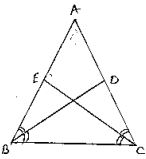Given that ΔABC is isosceles with AB = AC and BD and CE are bisectors of ∠B and ∠C
We have to prove BD = CE
Since, AB = AC
⇒ ∠ABC = ∠ACB  ...(1)
[∵ Angles opposite to equal sides are equal]
Since BD and CE are bisectors of ∠B and ∠C
⇒ ∠ABD = ∠DBC = ∠BCE = ECA = ∠B/2 = ∠C/2 ...(2)
Now,
Consider ΔEBC and ΔDCB
∠EBC = ∠DCB   [∵ ∠B = ∠C] from (1)
BC = BC  [Common side]